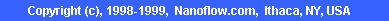Nanoflow Slit Rheometer Summary

 1. OverviewNanoflow slit rheometer is designed to measure the viscosity of thermoset polymers where the viscosity changes rapidly with time (such as epoxy molding compounds used in semiconductor-chip encapsulation). Unlike traditional viscometers, the Nanoflow rheometer can measure the viscosity at the actual processing ranges. Several tests using this rheometer show that the viscosity measured with this instrument is much more accurate than that from traditional rheometers.

 2. Advantages of the Nanoflow Slit Rheometer• The sample can be heated to the test temperature in a short time (2-3 seconds). Because of this fast heating, it can measure the viscosity of fast-curing thermosets (such as EMC).
• It is capable of measuring viscosity at typical processing ranges in chip encapsulation (in terms of shear rate, temperature and degree of cure). It can measure viscosity in shear rate of 10 - 5000, temperature of 100-180C and degree of cure of 0 - gel conversion.
• Because of the uniform distribution of temperature, shear rate and cure, and constant temperature and shear rate during testing, the viscosity as a function of shear rate, temperature and degree of cure can be measured accurately.
• It can measure the pressure dependence of viscosity.

 3. Verification of the Nanoflow Slit Rheometer• Spiral-flow lengths were calculated using the viscosity measured from the Nanoflow rheometer. In all cases tested so far, the calculated results are within 10% of the experimental results.
• Paddle-shift and wire-sweep values calculated using the Nanoflow rheometer viscosity data matched experimental results with about 10 - 20% of accuracy.

 4. Comparison with Other RheometersParallel-Plate Viscometer
• The viscosity is measured at low shear rates and low temperatures. To use this data for chip encapsulation analysis, the measured data have to be extrapolated to high shear rates and high temperatures. This extrapolation can lead to significant errors.
• For the spiral-flow cases tested so far, the calculated results using the parallel-plate data differed from the experimental results by 100 to 400%.
• Paddle-shift calculated using the parallel-plate rheometer viscosity data differed from the experimental results by about 800% for a test case.
Spiral-Flow Test Rheometer (RPA)
• The distribution of temperature, shear rate and cure is not uniform, and temperature and shear rate change during testing. This makes it difficult to measure the viscosity accurately.
• The parameters related to the viscosity dependence on temperature, shear rate and cure have to be determined inversely by numerical simulation. This can cause errors.
 5. Services